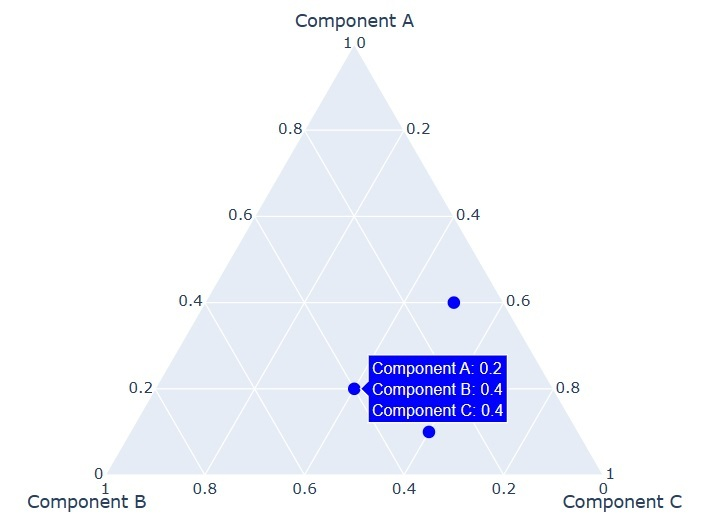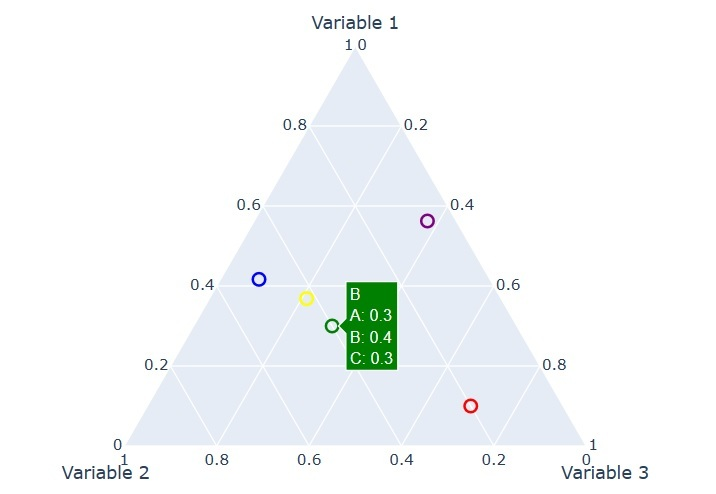# How to create Ternary Overlay using Plotly?

Ternary plots are a useful way to display compositional data where three variables add up to a constant value. Plotly is a powerful plotting library that can be used to create interactive ternary plots with ease. In this tutorial, we will explore how to create a Ternary Overlay using Plotty.

We are going to illustrate two examples to create an overlay using Plotly. By the end, we will learn the use Plotly to create stunning and informative ternary overlays. To create a Ternary Overlay using Plotly, we use the ‘scatterternary’ trace type. This trace type creates a scatter plot on a ternary diagram, where the components A, B, and C represent the vertices of an equilateral triangle. The position of the point within the triangle represents the proportion of each component in each data point.

## Example 1

Here’s an example of creating a Ternary Overlay using Plotly; here we use the ‘scatterternary’ trace type. This trace defines the lines connecting the vertices of the ternary plot. In this example, first, we created a scatter plot with three points on a ternary diagram with components A, B, and C. The ‘a’, ‘b’, and ‘c’ arrays represent the proportion of each component in each data point. The ‘marker’ dictionary defines the appearance of the markers on the plot. The ‘sum’ argument in the ‘ternary’ dictionary specifies that the three components should sum to 1. The ‘aaxis’, ‘baxis’, and ‘caxis’ dictionaries define the axis labels for the ternary diagram, next we created the figure in ‘fig’, and finally, we represented it by using ‘fig.show()’ fucntion.

import plotly.graph_objects as go

trace = go.Scatterternary(
a=[0.2, 0.4, 0.1],
b=[0.4, 0.1, 0.3],
c=[0.4, 0.5, 0.6],

mode='markers',
marker=dict(
symbol='circle',
color='blue',
size=10
)
)

layout = go.Layout(

ternary=dict(
sum=1,
aaxis=dict(title='Component A'),
baxis=dict(title='Component B'),
caxis=dict(title='Component C')
)
)

fig = go.Figure(data=[trace], layout=layout)
fig.show()


## Output## Example 2

In this example, first, the code produces a scatter plot on a ternary diagram with 5 points labelled A, B, C, D, and E. After then, each point is defined by its relative contribution to three variables, which are represented by the three vertices of the triangle. Next, the ‘sum’ parameter of the ‘ternary’ attribute is set to 1, meaning that each point's coordinates should sum to 1. Finally, we set the title using ‘title’ and the height to ‘500’; finally, we plotted it using the ‘fig.show()’ function.

import plotly.graph_objects as go
fig = go.Figure(go.Scatterternary({
'mode': 'markers',
'a': [0.1, 0.3, 0.5, 0.7, 0.9],
'b': [0.2, 0.4, 0.6, 0.8, 0.1],
'c': [0.7, 0.3, 0.1, 0.4, 0.6],
'marker': {
'symbol': 100,
'color': ['red', 'green', 'blue', 'yellow', 'purple'],
'size': 10,
'line': {'width': 2, 'color': 'white'}
},
'text': ['A', 'B', 'C', 'D', 'E']
}))

fig.update_layout({
'ternary': {
'sum': 1,
'aaxis': {'title': 'Variable 1'},
'baxis': {'title': 'Variable 2'},
'caxis': {'title': 'Variable 3'}
},
'height': 500
})

fig.show()


## OutputWe learned that a Ternary Overlay is a type of plot that displays data in a three-dimensional space using a triangle as the base, where the three corners of the triangle represent three variables whose sum is constant. Plotly is a popular Python library for creating interactive visualizations, including Ternary Overlays. It provides several functions to create an overlay; we can also customize the appearance of the plot by modifying the vertices and data lists and by adjusting the formatting of the traces and the layout of the figure using various Plotly options. Overall, Ternary Overlays can be useful for visualizing data with three components whose relative proportions are important, such as chemistry, geology, and ecology.

Updated on: 11-May-2023

151 Views